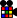# EPLL

(Redirected from EP)
 EPLLInformation Proposer(s): Proposed: Alt Names: Edges permutation of the last layer Variants: Beginner EP Subgroup: No. Algs: 4 Avg Moves: ~8.2 STM Purpose(s): Previous state: LL:EO cube state Next state: LL:EO+EP cube state
 The EPLL step is the step between the LL:EO cube state and the LL:EO+EP cube state.

Edges permutation of the last layer, abbrevaited EPLL, is the sub group of PLL where only the edges are permuted. EPLL is also used in many other methods, sometimes as a stand alone substep, like in COLL but also as a sub group, for example in ELL or ZBLL.

## EPLL algorithms

 Note that all of these algorithms are written in the Western notation, where a lowercase letter means a double-layer turn and rotations are denoted by x, y, and z. (how to add algorithms) Click on an algorithm (not the camera icon) to watch an animation of it.

### H Permutation

 Name: H-PLL, X-PLL Used in: EPLL, CPLL, PLL, ELL, ZBLL, ZZLL Optimal moves: 9 HTM, 7 STM X-PLL is H-PLL + U2.PLL M2' U M2' U2 M2' U M2'PLL M2' U' M2' U2' M2' U' M2'PLL R2' U2 R' U2 R2' U2' R2' U2 R' U2 R2'PLL M2' U2 M2' U' M2' U2 M2' UPLL M2' U2 M2' U M2' U2 M2' U'PLL R2 U2 R U2 R2 U2 R2 U2 R U2 R2PLL R2 U2 R2 U2 R2 U R2 U2 R2 U2 R2PLL R U2' R' U' R' U' R2 U' R2' U2' R2 U2' R' (U)PLL L2 U2 L' U2 L2 U2 L2 U2 L' U2 L2PLL L R U2 L' R' F' B' U2 F BPLL L R U2 L' R' (y) L' R' U2 L RPLL S R U2 R2 U2 R2 U2 R S'PLL F2 M2' F2 U' F2 M2' F2PLL (x) U2 M2' U2 B' U2 M2' U2 (x')PLL L R U2 L' l' U' u' L2 U (z) LPLL M2' u M2' u2 M2' u M2'PLL M2' u' M2' u2' M2' u' M2'

### U Permutation : a

 Name: U-PLL a Used in: EPLL, PLL, ELL, ZBLL, BLD Optimal moves: 9 HTM, 7 STMPLL R2 U' R' U' R U R U R U' RPLL (y2) R U' R U R U R U' R' U' R2PLL R U R' U R' U' R2 U' R' U R' U RPLL M2 U M' U2 M U M2PLL (y2) M2 U M U2 M' U M2PLL (y') M2 u' M u2 M u' M2PLL (y) M2 u' M' u2 M' u' M2PLL (y2) F2 U' (L R') F2 (L' R) U' F2PLL (y2) F2 U' M' U2 M U' F2PLL R U R' U' L' U' L U2 R U' R' U' L' U LPLL r U R' U R' U' R2 U' r' U R' U R

### U Permutation : b

 Name: U-PLL b Used in: EPLL, PLL, ELL, ZBLL, BLD Optimal moves: 9 HTM, 7 STMPLL R' U R' U' R' U' R' U R U R2PLL (y2) R2 U R U R' U' R' U' R' U R'PLL M2 U' M' U2 M U' M2PLL (y2) M2 U' M U2 M' U' M2PLL (y2) L' U L' U' L' U' L' U L U L2PLL (y') M2 u M' u2 M' u M2PLL R U' R U R U R' U' R' U' R' U2 R'PLL (y2) R' U' R U' R U R2 U R U' R U' R'PLL (y2) (L' U' L U) (R U R') U2 (L' U L) (U R U' R')PLL (z) U2' R U R U' R' U' R' U' R U' (z')PLL (y2 z) U' R U' R' U' R' U' R U R U2' (z')PLL (y2) F2 U M' U2 M U F2PLL (y') r2 u M' u2 M' u r2PLL (y2) (L' U' L U' L' U2 L) U' (R U R' U R U2' R')

### Z Permutation

 Name: Z-PLL Used in: EPLL, PLL, ELL, ZBLL, ZZLL Optimal moves: 12 HTM, 7 STMPLL M2 U M2 U M U2 M2 U2 M U2PLL M2 U' M (U2 M2 U2) M U M2PLL R' U' R2 U R U R' U' R U R U' R U' R'PLL R U R' U R' U' R' U R U' R' U' R2 U RPLL (y) (M2' U')2 M' (U2' M2' U2') M'PLL M2' U2 M' U' M2' U' M2' U' M'PLL (y) M2' U2 M' U M2' U M2' U M'PLL M' U' M2' U' M2' U' M' U2 M2'PLL (y) M' U M2' U M2' U M' U2 M2'PLL M' U2 M2 U2 M' U' M2 U' M2PLL M2' U M2' U' E2 M' E2 M'PLL (y) M2' U' M2' U E2 M' E2 M'PLL M2' U M' E2 M' E2 U' M2'PLL M2' U M2' U M2 B2 M2 B2PLL (M2' U)2 M' (U2 M2' U2) M' U2PLL U R' U' R U' R U R U' R' U R U R2 U' R' UPLL (y) R U R2 U' R' U' R U R' U' R' U R' U RPLL (x') R U' R' (U D) R' (D U') R' U R D2' (x)PLL M2' u M2' D' M S2 M'PLL (y) R2 U R2 U' R2 F2 R2 U' F2 U R2 F2PLL R2 U' R2 U R2 (x') U2 R2 F U2 F' R2 U2 (x)PLL (y') l' U R U' D' R U D' R U' R' D2 (x')PLL R' U' R' F R F' U R F' U' L' U L FPLL R' U' R' F R F' U R U' R' U' F' U F RPLL R U R2 U' R' F R U R U' R U' R' U' R U R' F'